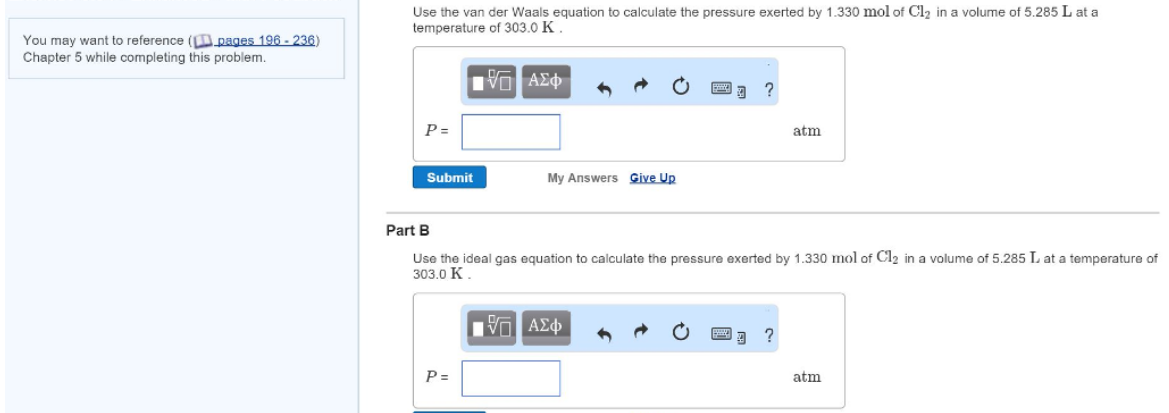# Problem: Use the van der Waals equation to calculate the pressure exerted by 1.330 mol of Cl_2 in a volume of 5.285 L at a temperature of 303.0 K. Use the ideal gas equation to calculate the pressure exerted by 1.330 mol of Cl_2 in a volume of 5.285 L at a temperature of 303.0 K.

###### FREE Expert Solution
89% (45 ratings)###### Problem Details

Use the van der Waals equation to calculate the pressure exerted by 1.330 mol of Cl_2 in a volume of 5.285 L at a temperature of 303.0 K.

Use the ideal gas equation to calculate the pressure exerted by 1.330 mol of Cl_2 in a volume of 5.285 L at a temperature of 303.0 K.